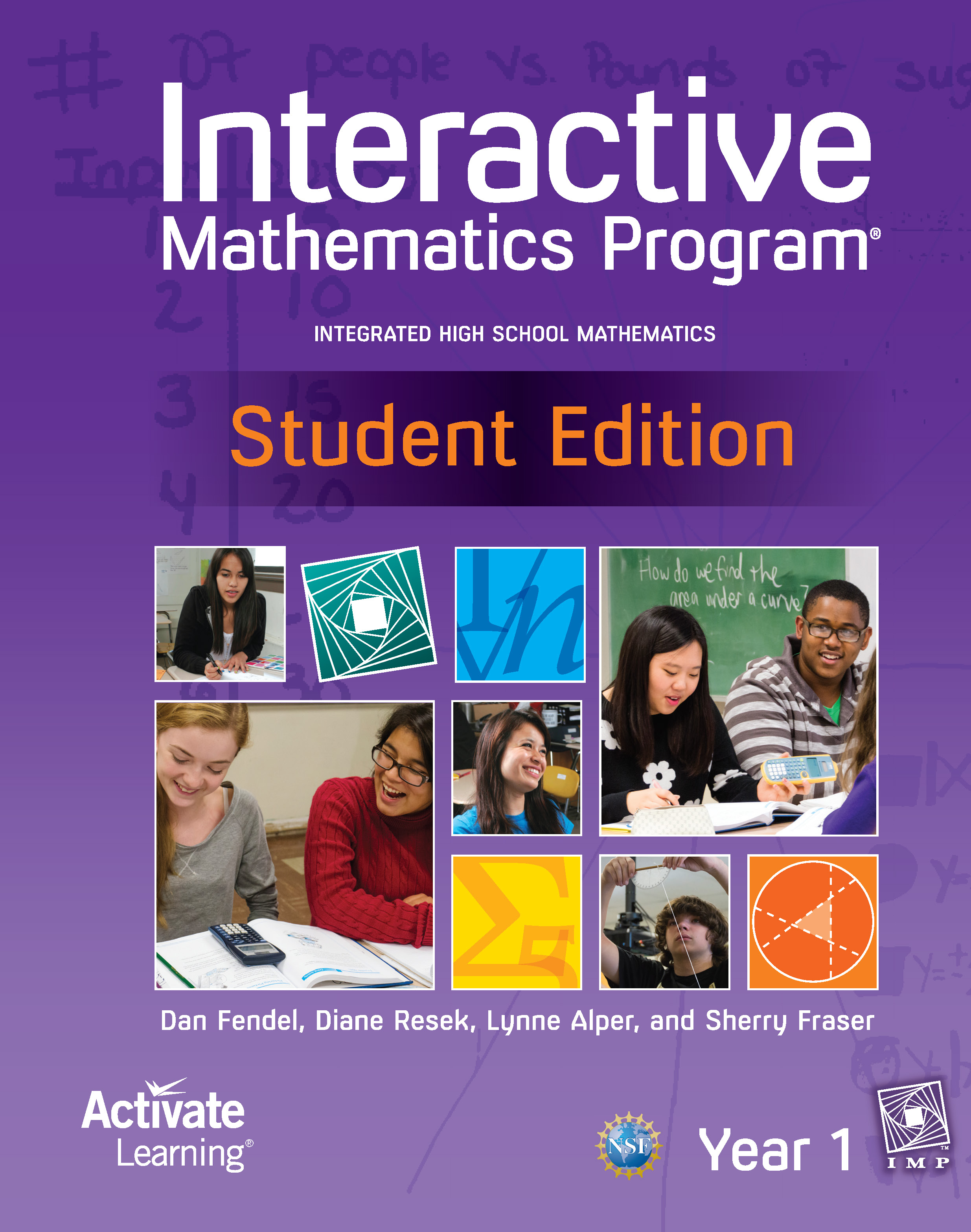# Interactive Mathematics Program

Introducing IMP®: No other curriculum embeds the CCSSM practices and standards so deeply. Interactive Mathematics Program is an integrated high-school mathematics curriculum designed to challenge students with a four-year sequence of college-preparatory mathematics. The curriculum has demonstrated in schools throughout the country that the successful study of advanced mathematics is an achievable standard for ALL students.

Interactive Mathematics Program® is an “exemplary” math curriculum.
IMP is one of three comprehensive high-school mathematics curricula identified as “Exemplary” by the U.S. Department of Education for providing convincing evidence of its effectiveness in multiple schools with diverse populations.

Interactive Mathematics Program places a strong emphasis on mathematical reasoning.
In IMP, students rely on mathematical reasoning to solve challenging problems, based on real-world situations as well as meaningful scenarios. With an emphasis on critical thinking, students develop multiple strategies to solve problems.

Interactive Mathematics Program® is technology-enhanced.
The IMP curriculum incorporates graphing calculators as an integral part of the development of mathematical ideas. The calculators enable students to see mathematics and problem solving in a different way and allow them to focus on ideas.